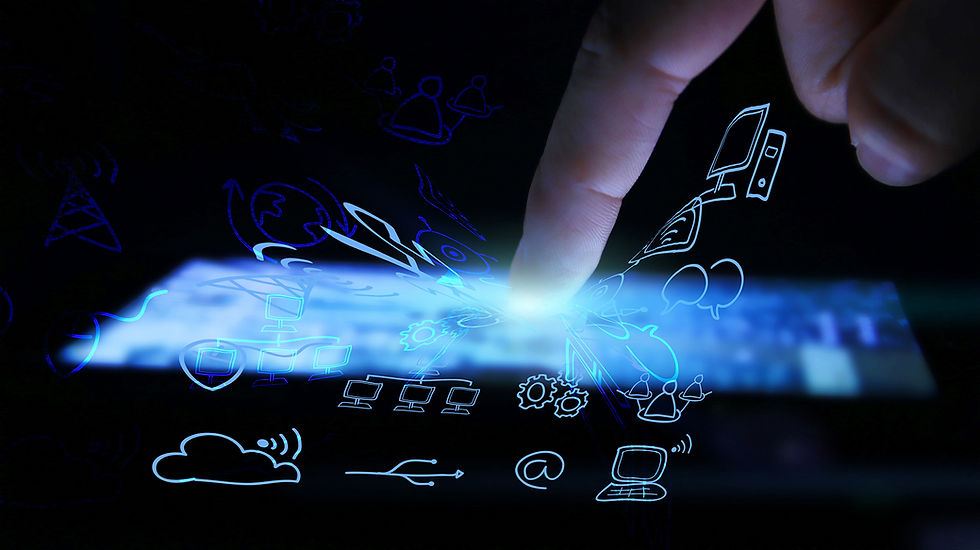top of page
Blog: Blog2
Search
• Quantum AI

# This Year's Cloud Computing Technology Trends

Gordon Moore is one of the Intel co-founders.  In 1965, Gordon Moore made a prediction by observing emerging trend that computing would increase power and decrease relative cost. He made assumption that the number of transistors per square inch on integrated circuits will double every year since the integrated circuit was invented. But, this law is now believed to be the last till 2020.

The computer processing power depends on the number of transistors used. Transistor is a type of switch which can be respond to either binary 1 or binary 0. The group of transistors into special circuits which are called logic gates allows the computer to perform calculations and make decisions according to man-made computer program. Today, where a typical scale for transistors is 14nm, it has reached to the size of an atom. The 22 core Xeon E5 processors has over 7.2 billion transistors.  But as it is reaching out to its physical limits we are approaching a real physical barrier for our technological progress. So, what after this? To solve this problem, scientist are trying to use quantum properties in building quantum computers.Yuri Manin, the Russian physicist and mathematician was the first to propose the idea of quantum computing in 1980. Quantum technology is based on quantum mechanical phenomena. At the present scenario quantum computing is based on binary, the data is encoded into binary digits 'Bits', can be of two definite state 0 or 1, true or false, on or off. Quantum computing uses Quantum bits (Qubits) which can be of any state 0 and 1 simultaneously.  Quantum computers work with qubits that are represented by the quantum mechanical states of subatomic particles.  Qubit uses two key principals of quantum physics, superposition and entanglement. Superposition means that each qubit can represent 0 or 1 or both at the same time, and entanglement happen when two qubits in a superposition are correlated with one another meaning a state of one whether it’s a 0 or 1 or both depends on the state of another. Using these two principles qubits can act as a much more sophisticated versions of switches, helping quantum computer companies solve difficult problem that are impossible with classic computers.

For example: 2 bits’ data system 0 and 1, will be of four different possible state 00, 01, 10, 11, so computer has to perform four operations to analyze or process data or information. 2 qubit examine all of these possibilities at same time in one operations. So 4 qubit computer could evaluate 16 state in one single operation. In comparison with 4 bits’, computer can analyze only one state in single operation, so it has to repeat the operation for 16 times to process the data.

#TheCloud #Trends #2018